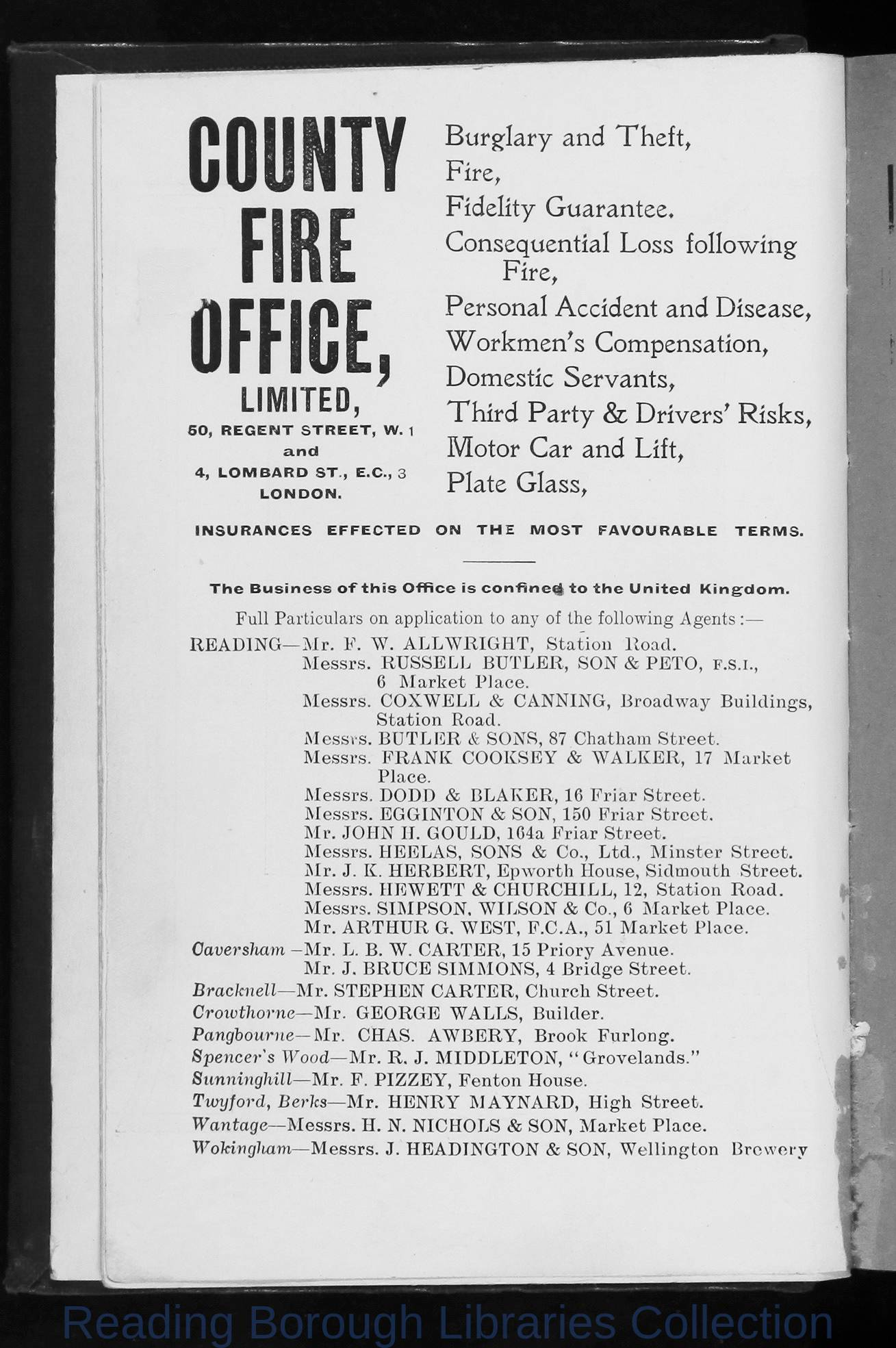## Image Details

There is no information available.

## OCR Text

COUNTY
FIRE
OFFICE,

Burglary and Theft,
Fire,
Fidelity Guarantee.
Consequential Loss following
Fire,
Personal Accident and Disease,
W orkmenâ s Compensation,
Domestic Servants,
Third Party & Drivers' Risks,
Motor Car and Lift,
Plate Glass,

L IM IT E D ,
6 0 , R E G E N T S T R E E T , W. 1
and
4, L O M B A R D S T . , E . C ., 3
LONDON.
IN S U R A N C E S

EFFECTED

ON

TH E

M OST

FAVOURABLE

T h e B u s i n e s s o f t h i s O f f i c e is c o n f i n e ^ t o t h e U n i t e d

TERM S.

K in g d o m .

F u l l P a r t i c u l a r s o n a p p l i c a t i o n t o a n y o f t h e f o l l o w i n g A g e n t s :â
R E A D IN G â M r.

F .

M essrs.

W . A L L W R IG H T ,
R U S S E L L
6

M essrs.

M a rk e t

S ta tio n

&

&

C A N N IN G ,

P E T O ,

f

B ro a d w a y

. s . i .,
B u ild in g s ,

F R A N K

M essrs. D O D D

S O N

P la c e .

C O X W E L L

M essrs. B U T L E R
M essrs.

S ta tio n

B U T L E R ,

&

& S O N S , 87 C h a th a m
C O O K S E Y

&

B L A K E R , 16

M e ssrs. E G G IN T O N

&

S tre e t.

W A L K E R ,

17

M a rk e t

F r ia r S t r e e t.

S O N , 15 0

F r ia r S t r e e t.

M r . J O H N IT . G O U L D , 1 6 4 a F r i a r S t r e e t ,
M essrs. H E E L A S,

SO N S

&

C o .,

M r. J. K . H E R B E R T , E p w o r th
M essrs. IIE W E T T

&

M essrs. S IM P S O N , W IL S O N
M r. A R T H U R

Caversham

L t d .,

M in s te r

H o u s e , S id m o u th

C H U R C H IL L , 12, S ta tio n
&

C o ., 6

S tre e t.
S tre e t.

M a r k e t P la c e .

G . W E S T , F .C .A ., 5 1 M a r k e t P la c e .

- M r . L . B . W . C A R T E R , 15 P r io r y A v e n u e .
M r. J. B R U C E

S IM M O N S , 4 B r id g e S tre e t.

Bracknell â M r . S T E P H E N C A R T E R , C h u r c h S t r e e t .
Crowthorne â M r . G E O R G E W A L L S , B u i l d e r .
Pangbourneâ M r . C H A S . A W B E R Y , B r o o k F u r l o n g .
Spencer's Woodâ M r . R . J . M I D D L E T O N , â G r o v e l a n d s . â
Sunninghillâ M r . F . P I Z Z E Y , F e n t o n H o u s e .
Tivyford , B e r k s â M r . H E N R Y M A Y N A R D , H i g h S t r e e t .
Wantage â M e s s r s . H . N . N I C H O L S & S O N , M a r k e t P l a c e .
Wokingham â M e s s r s . J . H E A D I N G T O N & S O N , W e l l i n g t o n

B rew ery